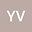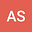THE CLASS OF EVOLUTIONARY FERRODYNAMIC EQUATIONS
••• Yuri VIRCHENKO,
• Andrey Subbotin
Yuri VIRCHENKO
Belgorod National Research University

Corresponding Author:[email protected]

Author ProfileAndrey Subbotin
Belgorod National Research University
Author Profile## Abstract

A linear manifold ${\mathcal K}_2$ of evolutionary equations for a pseudovector field on ${\Bbb R}^3$ is described. An infinitisimal shift of each equation is determined by a second-order differential operator of divergent type. All operators are invariant with respect to space translations in ${\Bbb R}^3$, relative to time translations, and they are transformed by covariant way relative to rotations of ${\Bbb R}^3$. It is proved that the linear space ${\mathcal M}_2 \subset {\mathcal K}_2$ of differential operators preserving solenoidal property and unimodularity of the field is one-dimensional and an explicit form of such operators is found.
17 Jan 2020Submitted to Mathematical Methods in the Applied Sciences
18 Jan 2020Submission Checks Completed
18 Jan 2020Assigned to Editor
27 Jan 2020Reviewer(s) Assigned
08 May 2020Review(s) Completed, Editorial Evaluation Pending
11 May 2020Editorial Decision: Revise Major